Journal DetailsFormat
Journal
eISSN
2354-0192
First Published
28 Dec 2018
Publication timeframe
1 time per year
Languages
EnglishOpen Access

# Numerical study of coupled oscillator system using the classical Euler-Lagrange equations

###### Accepted: 08 Dec 2020
Journal DetailsFormat
Journal
eISSN
2354-0192
First Published
28 Dec 2018
Publication timeframe
1 time per year
Languages
English

Problems involving vibrations (mechanical or electrical) can be reduced to problems of coupled oscillators. For this, we consider the motion of coupled oscillators system using Lagrangian method. The Lagrangian of the system was initially constructed, and then the Euler-Lagrange equations (i.e., equations of motion of the system) have been obtained. The obtained equations of motion are a homogenous second-order equation. These equations were solved numerically using the ode45 code, which is based on Runge-Kutta method.

#### Keywords

Introduction

In classical mechanics, there are many useful techniques that can be used in solving physical systems. Newtonian mechanics is a technique based on vector concepts (i.e., forces). In this technique, one has to write down all forces acting on the system, and then, applying Newton’s second law. Another useful technique based on scalars quantities (i.e., kinetic and potential energies) has been developed. This technique, known as Lagrangian mechanics, helped physicists in solving a large number of complicated systems, where Newtonian techniques cannot be applied effectively in many areas in physics, such as classical mechanics [1, 2], nonlinear systems, and control theory [3, 4].

To apply Lagrangian mechanics, one has to write down the kinetic and potential energies of the system, and as a result, the Lagrangian L(, q, t) is obtained, where q represents the generalized coordinate, is the generalized velocity, and t is the time. Applying the following equation $∂L∂q−ddt∂L∂q˙=0$ {{\partial L} \over {\partial q}} - {d \over {dt}}{{\partial L} \over {\partial \dot q}} = 0 to the Lagrangian L(, q, t), we obtained a set of differential equations known as Euler–Lagrange equations (ELE) or equations of motion. For more details, one can refer to . The ELE can be solved analytically, and thus, the motion of the system is specified. In many cases, the ELE cannot be solved analytically. Therefore, we have to seek for suitable numerical techniques to apply, and in this case, some initial conditions are needed.

On the other hand, it is important to remember that all mechanical and electrical vibration problems reduce, in the case of small oscillations, to problems involving one or several coupled oscillators. Problems involving vibrations of strings, membranes, elastic solids, electrical, or acoustical can be reduced to problems of coupled oscillators; see for examples [5, 6] and references within them.

Many numerical methods have been introduced in literature to solve differential equations [7, 8, 9, 10, 11, 12]. These numerical methods are based on MATLAB, MATHEMATICA, MATCAD software and other computerized programs. For example, MATLAB contains many built-in codes that can be used in such cases (e.g., ode45). These numerical techniques enable us to solve many systems numerically, even when the analytical solution is not known. In addition, the ode45 code depends on a Runge-Kutta method, which can be used efficiently in solving different kinds of differential equations. One can refer to [13, 14] and references therein to construct a good idea about applying the Runge-Kutta method on solving differential equations.

On the other hand, many ideas have been carried out by applying MATLAB and built-in codes in solving some differential equations. For example in [15, 16, 17], the authors solved some differential equations describing physical systems by applying Lagrangian mechanics and obtaining the ELEs, which are in general differential equations that need to be solved numerically for certain initial conditions.

The outline of this paper is arranged as follows: In Section 2, the physical description of the system is presented, and the Lagrangain of the coupled oscillator is obtained. In Section 3, the numerical method used is presented. Section 4 provides the numerical solutions of the derived equations of motion for different initial conditions. The results were discussed in Section 5. We finally end the paper with conclusions.

Physical description of the coupled oscillator

Let us consider the system shown in Figure 1, which consists of two coupled carts of masses m1 and m2, attached to their respective walls by two identical springs (with force constant k) and to each other by another spring (with force constant k2) . It is of interest to mention that in some books, this system is known as: diatomic molecules.

Assuming all springs to be massless, we aim to obtain the ELE for the system, and then we require obtaining a numerical solution for these equations under different initial conditions.

First of all, the kinetic energy (T) of the system reads: $T=12m1x˙12+12m2x˙22.$ T = {1 \over 2}{m_1}\dot x_1^2 + {1 \over 2}{m_2}\dot x_2^2.

In order to write the potential energy (V) of the system, we have to identify the extensions of the three springs shown in Figure 1 as (x1,x1x2, and −x2). As a result, the potential energy reads: $V=12(k+k2)x12−k2x1x2+12(k2+k)x22.$ V = {1 \over 2}\left({k + {k_2}} \right)x_1^2 - {k_2}{x_1}{x_2} + {1 \over 2}\left({{k_2} + k} \right)x_2^2.

The classical Lagrangian can be immediately written as: $L=T−V=12m1x˙12+12m2x˙22−12(k+k2)x12+k2x1x2−12(k2+k)x22.$ \matrix{L \hfill & {= T - V = {1 \over 2}{m_1}\dot x_1^2 + {1 \over 2}{m_2}\dot x_2^2 - {1 \over 2}\left({k + {k_2}} \right)x_1^2} \hfill \cr {} \hfill & {+ {k_2}{x_1}{x_2} - {1 \over 2}\left({{k_2} + k} \right)x_2^2.} \hfill \cr}

Our final step in this section is in obtaining the equations of motion. As such, applying our Lagrangian (eq. 3) to the following equation $∂L∂q−ddt∂L∂q˙=0$ {{\partial L} \over {\partial q}} - {d \over {dt}}{{\partial L} \over {\partial \dot q}} = 0 , with q = x1, x2, we got the following ELE:

For q = x1, we have: $m1x¨1=−(k−k2)x1+k2x2.$ {m_1}{\ddot x_1} = - \left({k - {k_2}} \right){x_1} + {k_2}{x_2}.

While for q = x2, we have: $m2x¨2=−k2x1+(k2+k)x2.$ {m_2}{\ddot x_2} = - {k_2}{x_1} + \left({{k_2} + k} \right){x_2}.

Now, we aim to obtain the Hamilton’s equations of motion. The first point is introducing the following generalized momenta: $px1=∂L∂x˙1=mx˙1.$ {p_{{x_1}}} = {{\partial L} \over {\partial {{\dot x}_1}}} = m{\dot x_1}. $px2=∂L∂x˙2=mx˙2.$ {p_{{x_2}}} = {{\partial L} \over {\partial {{\dot x}_2}}} = m{\dot x_2}.

As a result, the Hamiltonian H = ∑ ipxiL, with I = 1, 2 reads: $H=px122m1+px222m1+12(k+k2)x12−k2x1x2+12(k2+k)x22.$ \matrix{H \hfill & {= {{p_{{x_1}}^2} \over {2{m_1}}} + {{p_{{x_2}}^2} \over {2{m_1}}} + {1 \over 2}\left({k + {k_2}} \right)x_1^2 - {k_2}{x_1}{x_2}} \hfill \cr {} \hfill & {+ {1 \over 2}\left({{k_2} + k} \right)x_2^2.} \hfill \cr}

Finally, the Hamilton’s equations can be obtained as: $x˙1=∂H∂Px1⇒m1x¨1=−(k+k2)x1+k2x2.$ {\dot x_1} = {{\partial H} \over {\partial {P_{{x_1}}}}} \Rightarrow {m_1}{\ddot x_1} = - \left({k + {k_2}} \right){x_1} + {k_2}{x_2}. $x˙2=∂H∂Px2⇒m2x¨2=k2x1−(k2+k)x2.$ {\dot x_2} = {{\partial H} \over {\partial {P_{{x_2}}}}} \Rightarrow {m_2}{\ddot x_2} = {k_2}{x_1} - \left({{k_2} + k} \right){x_2}.

In the section below, we aimed to obtain numerical solution for eqs. (9, 10) for some initial conditions.

Numerical solution method

A standard solver for ordinary differential equations (ODEs) in the simulation of MATLAB’s framework is the ode45 function. ODE45 executes the Runge-Kutta method with a variable time step for optimal computation [18, 19, 20]. A fourth-order Runge-Kutta algorithm is useful in solving non-linear differential equations, taking into consideration some given initial conditions . Any problem in the following manner $dxdt=f(t,x).$ {{dx} \over {dt}} = f\left({t,x} \right).

With x(to) = xo

Where t is the independent variable, x is a vector of dependent variables to be found, and f (t, x) is a function of t, and x can be solved using ode45. Specifying f (t, x) and the initial conditions enable us to solve the problem .

Bellow a short explanation is presented to explain how Runge-Kutta works .

In general, given that: $y'=f(x,y).$ y' = f\left({x,y} \right). $y(xn)=yn.$ y\left({{x_n}} \right) = {y_n}.

Then, one can compute in turn: $k1=hf(xn,yn).$ {k_1} = hf\left({{x_n},{y_n}} \right). $k2=hf(xn+h2,yn+k12).$ {k_2} = hf\left({{x_n} + {h \over 2},{y_n} + {{{k_1}} \over 2}} \right). $k3=hf(xn+h2,yn+k22).$ {k_3} = hf\left({{x_n} + {h \over 2},{y_n} + {{{k_2}} \over 2}} \right). $k4=hf(xn+h,yn+k3).$ {k_4} = hf\left({{x_n} + h,{y_n} + {k_3}} \right). $yn+1=yn+16(k1+2k2+2k3+k4).$ {y_{n + 1}} = {y_n} + {1 \over 6}\left({{k_1} + 2{k_2} + 2{k_3} + {k_4}} \right).

Now, everything becomes in first-order form, so the list of commands is needed in the code .

ODE45 is used to implement “first try” solution for most problems [25, 26]. For more details about the Runge-Kutta method, one can refer to [23, 27].

Repetition and conditional structures are used to repeat sequences in MATLAB, and from these structures are: if and if-else statements, do while, for each, while, and for loops.

In literature, there are many examples of repetition and conditional structures [23, 27].

In Section 4, we propose the numerical solution for eqs. (4 and 5) using loop structure. We consider some initial conditions, and the numerical solution for these particular conditions has been obtained.

Simulation results

Below, we consider some selected cases to obtain the numerical solution of eqs. (4, 5):

Case 1: Symmetric Case

In this case, we considered the two initial conditions x1(0) = −x2(0) = 1 and 1(0) = − 2(0) = 0.5; on the other hand, the other parameters were assumed to be k = 1, 2, with k2 = 2k, while m1 = 3 and m2 = 7, see Figures 2, 3: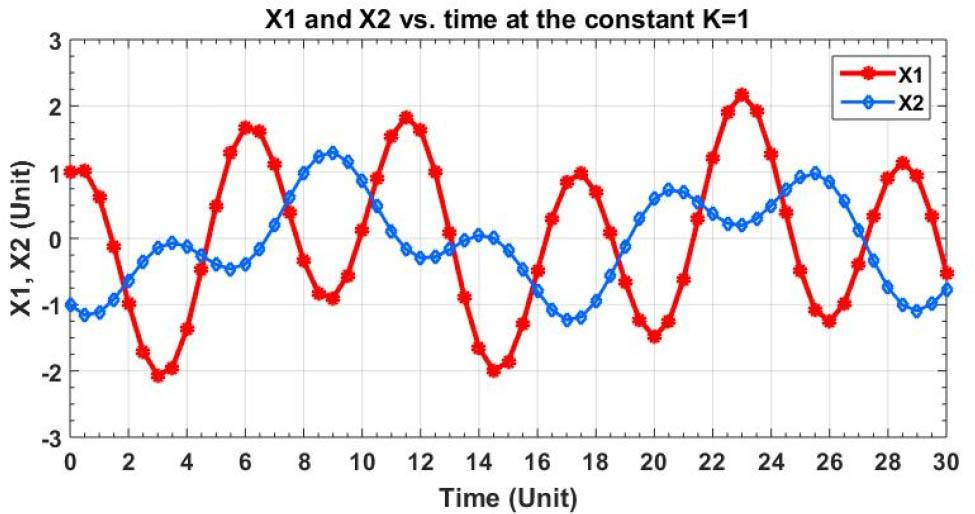The positions x1 and x2 of masses m1 and m2, respectively, against time t (the red line for mass m1, and the black line for mass m2).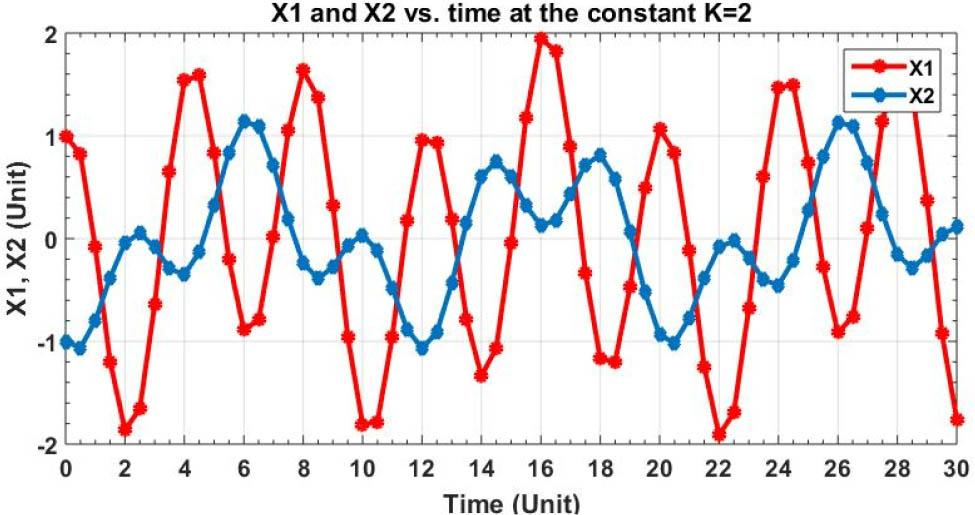The positions x1 and x2 of masses m1 and m2, respectively, against time t (the red line for mass m1 and the black line for mass m2).
Case 2: Anti-symmetric Case

In this case, we considered the following two initial conditions x1(0) = x2(0) = 1 and 1(0) = 2(0) = 0.5, and the other parameters were assumed to be k = 1, and 2, with k2 = 2k, while m1 = 3 and m2 = 7, see Figures 4, 5: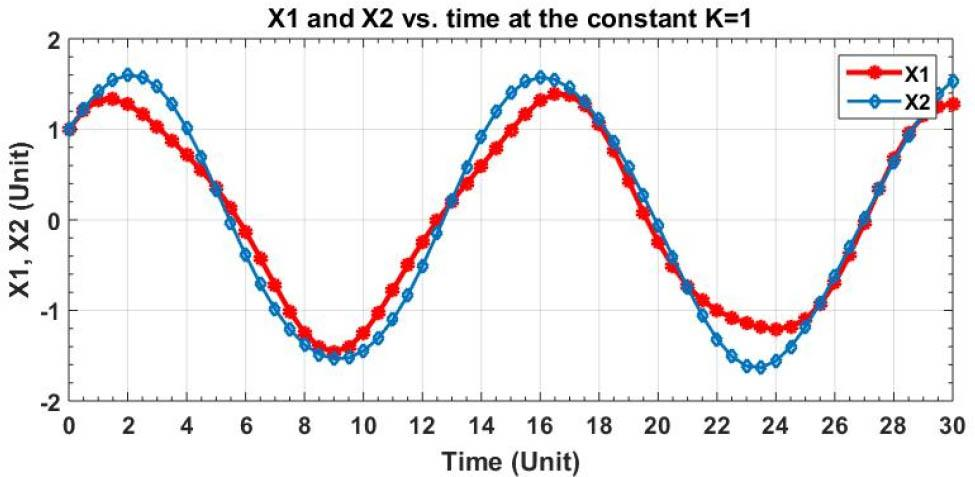The positions x1 and x2 of masses m1 and m2, respectively, against time t (the red line for mass m1 and the black line for mass m2).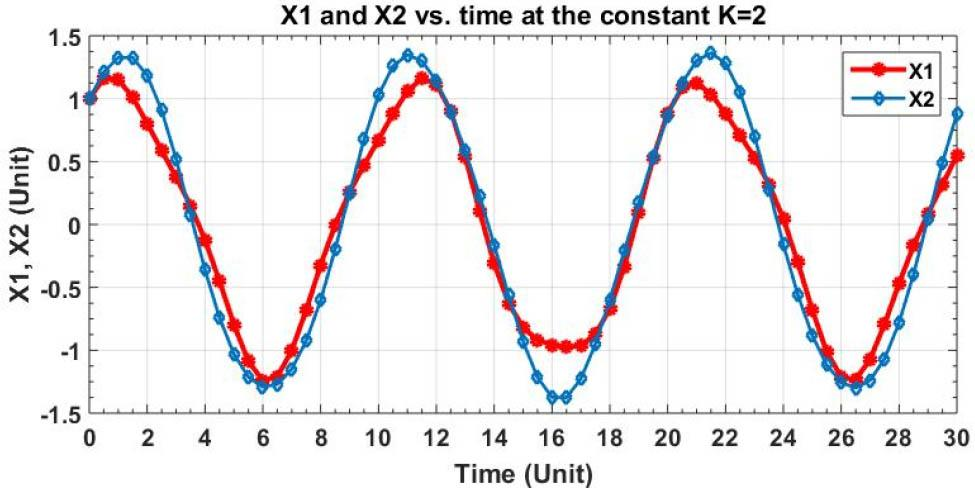The positions x1 and x2 of masses m1, and m2, respectively, against time t (the red line for mass m1 and the black line for mass m2).
Case 3: Weak Coupled Oscillator (k2 ≺≺ k)

Finally, we considered the weak coupled oscillator case, which is of special importance. In this case, the following parameters are considered k = 1, and 2, with k2 = 0.01, while m1 = 3 and m2 = 7, with the following two initial conditions x1(0) = 1, x2(0) = 0 and 1(0) = 2(0) = 0, see Figures 6, 7: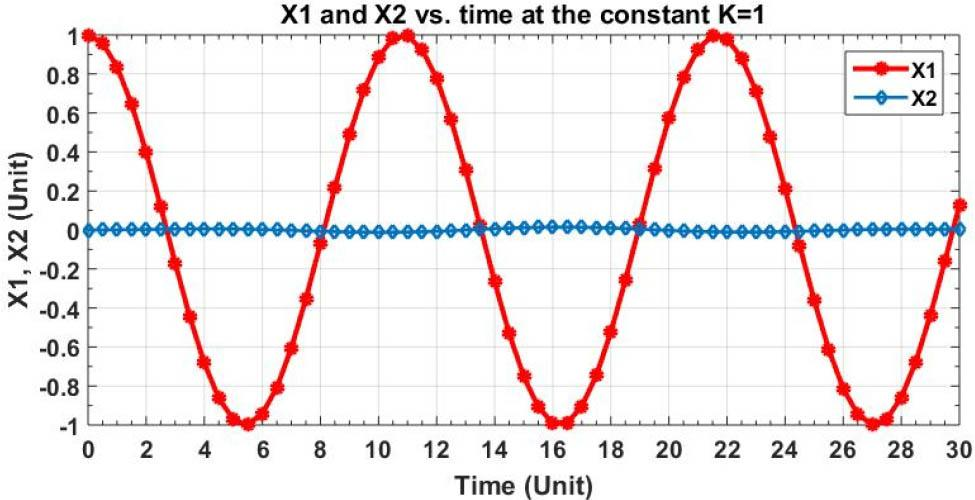The positions and x2 of masses m1, and m2, respectively against time t (the red line for mass m1, and the black line for mass m2).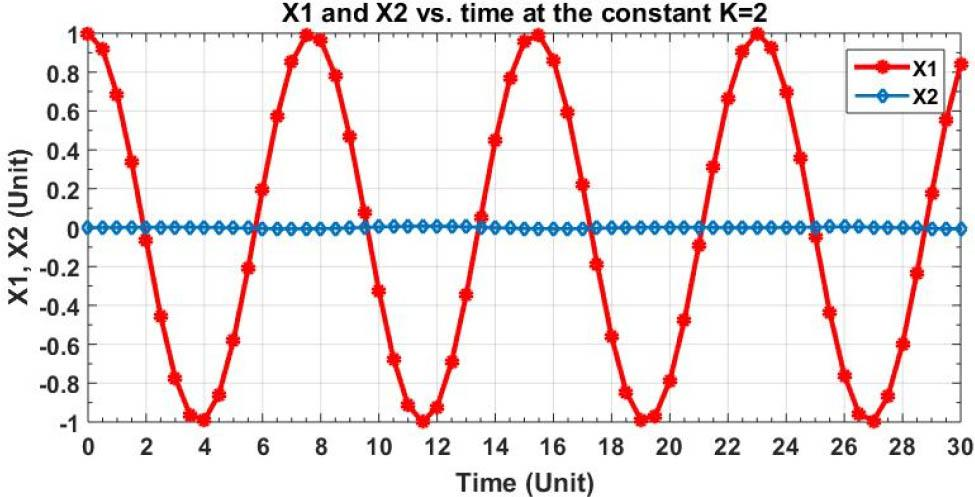The positions x1 and x2 of masses m1 and m2, respectively against time t (the red line for mass m1 and the black line for mass m2).
Results and Discussion

In this paper, the motion of the coupled oscillator system has been studied numerically using the ode45 code, and the solutions x1(t) and x2(t) have been plotted for the several considered cases as in Figures 27.

Figures 2, 3 present the positions x1(t) and x2(t) of masses m1 and m2 against the time, respectively, for the symmetric case. The results in the same figures show that the periodicity of the oscillations has disappeared. Also, the influence on mass m2 is clearer than that on m1, because it has a larger value. In addition, because m1 and m2 were displaced initially in opposite directions (i.e., x1(0) = −x2(0)) and then released, this is the main reason for disappearing the periodicity of the oscillations.

Figures 4, 5 present the positions x1(t) and x2(t) of masses 1 and 2 against the time, respectively, for the antisymmetric case. Again, the periodicity of the oscillation has disappeared, but less than that in Figures 2, 3. The reason here refers to the fact that the two masses m1 and m2 were displaced initially in the same direction (i.e., x1(0) = x2(0)) and then released.

For Cases 1 and 2, it is expected that the periodicity of the oscillations will be destroyed. This is due to the fact that the spring-connected the two masses together have a larger stiffness than the two other springs.

In Figures 6, 7, the positions x1(t) and x2(t) of masses 1 and 2, respectively, is displaced against time for the weak coupled oscillator, where the stiffness of the spring connecting the two masses is very small compared with the other springs stiffness. In our case, we consider k2 = 0.01, whereas k = 1 is shown in Figure 6, and k = 2 is shown in Figure 7. It is clear from these two figures that mass m1 undergoes a simple harmonic oscillations, with a constant amplitude and a constant period, while mass m2 nearly vibrates but with very small oscillations around the equilibrium position; this is due to the fact that m2 has a larger value than m1.

Finally, one can see from the figures that if the stiffness of the spring connecting the two masses is larger than the other springs’ stiffness, then this will not allow the system to undergo simple harmonic motion. As a result, the periodicity of the motion is destroyed.

Conclusion

In conclusion, the periodicity of the oscillator disappeared, especially in the symmetric case. While in the antisymmetric case, the periodicity is nearly still there and not destroyed. In the weak coupled case, one can see from Figures 6, 7 that mass m2 does not move, because it is larger than m1.

After releasing the system, the displacement in the symmetric and anti-symmetric cases exceeds the initial displacement. This can be explained by the effects of springs on each other. Nonetheless, for the weak coupled oscillator, mass m1 undergoes a simple harmonic motion and not affected by mass m2.

Marion J. B., and Thornton S. T. Classical Dynamics of Particles and Systems, 3rd edn. (Harcourt Brace Jovanovich), 1988. MarionJ. B. ThorntonS. T. Classical Dynamics of Particles and Systems 3rd edn. Harcourt Brace Jovanovich 1988 Search in Google Scholar

Hand L. N., and Finch J. D. Analytical Mechanics. Cambridge University Press, 2012. HandL. N. FinchJ. D. Analytical Mechanics Cambridge University Press 2012 Search in Google Scholar

Murray R. M. Nonlinear control of mechanical systems: A Lagrangian perspective. Annual Reviews in Control. Vol. 21, pp. 31–42, 1997. MurrayR. M. Nonlinear control of mechanical systems: A Lagrangian perspective Annual Reviews in Control 21 31 42 1997 10.1016/B978-0-08-042371-5.50063-0 Search in Google Scholar

Musielak Z. E., Roy D., and Swift L. D. Method to derive Lagrangian and Hamiltonian for a nonlinear dynamical system with variable coefficients. Chaos, Solitons and Fractals. Vol. 38, no. 3, pp. 894–902, 2008. MusielakZ. E. RoyD. SwiftL. D. Method to derive Lagrangian and Hamiltonian for a nonlinear dynamical system with variable coefficients Chaos, Solitons and Fractals 38 3 894 902 2008 10.1016/j.chaos.2007.06.076 Search in Google Scholar

Hajimiri A., and Lee T. A General Theory of Phase Noise in Electrical Oscillators,” IEEE Solid State Circuits, vol. 33, no. 2, pp. 179–194, 1998. HajimiriA. LeeT. A General Theory of Phase Noise in Electrical Oscillators” IEEE Solid State Circuits 33 2 179 194 1998 10.1109/4.658619 Search in Google Scholar

Liu M. L. Principle and Application of Oscillator, Higher Education Press, Beijing, pp. 41–43, 1984. LiuM. L. Principle and Application of Oscillator Higher Education Press Beijing 41 43 1984 Search in Google Scholar

Butcher J. C. Numerical methods for ordinary differential equations, second revised ed., Wiley, Chichester, 2008. ButcherJ. C. Numerical methods for ordinary differential equations second revised ed. Wiley Chichester 2008 10.1002/9780470753767 Search in Google Scholar

Hairer E., Lubich C., and Wanner G. Geometric numerical integration. Structure preserving algorithms for ordinary differential equations. 2nd revised ed., Springer, Berlin, 2006. HairerE. LubichC. WannerG. Geometric numerical integration. Structure preserving algorithms for ordinary differential equations 2nd revised ed. Springer Berlin 2006 Search in Google Scholar

Deuflhard P., and Bornemann F. Scientific computing with ordinary differential equations, Springer, New York, 2002. DeuflhardP. BornemannF. Scientific computing with ordinary differential equations Springer New York 2002 10.1007/978-0-387-21582-2 Search in Google Scholar

Atkinson K., Han W., and Stewart D. Numerical Solution of Ordinary Differential Equations. A John Wiley and sons, Inc., Publication, 2008. AtkinsonK. HanW. StewartD. Numerical Solution of Ordinary Differential Equations A John Wiley and sons, Inc., Publication 2008 10.1002/9781118164495 Search in Google Scholar

Potra F. A., and Yen J. Implicit Numerical Integration for Euler-Lagrange Equations via Tangent Space Parameterization. Mechanics of Structures and Machines, vol. 19, no. 1, pp. 77–98, 2007. PotraF. A. YenJ. Implicit Numerical Integration for Euler-Lagrange Equations via Tangent Space Parameterization Mechanics of Structures and Machines 19 1 77 98 2007 10.1080/08905459108905138 Search in Google Scholar

Rheinboldt W. C. Performance Analysis of Some Methods for Solving Euler-Lagrange Equations. Appl. Math. Lett. vol. 8, no. 1, pp. 77–82, 1995. RheinboldtW. C. Performance Analysis of Some Methods for Solving Euler-Lagrange Equations Appl. Math. Lett. 8 1 77 82 1995 10.1016/0893-9659(94)00114-R Search in Google Scholar

Hussain K. A., Ismail F., Senu N., and Rabiei F. Fourth-Order Improved Runge-Kutta Method for Directly Solving Special Third-Order Ordinary Differential Equations. Iranian Journal of Science and Technology, Transaction A: Science. Vol. 41, no. 2, 2017. HussainK. A. IsmailF. SenuN. RabieiF. Fourth-Order Improved Runge-Kutta Method for Directly Solving Special Third-Order Ordinary Differential Equations Iranian Journal of Science and Technology, Transaction A: Science 41 2 2017 10.1007/s40995-017-0258-1 Search in Google Scholar

Chauhan, V., Srivastava, P.K. Computational techniques based on Runge-Kutta method of various order and type for solving differential equations. International Journal of Mathematical, Engineering and Management Sciences Vol. 4, no. 2, 2019. ChauhanV. SrivastavaP.K. Computational techniques based on Runge-Kutta method of various order and type for solving differential equations International Journal of Mathematical, Engineering and Management Sciences 4 2 2019 10.33889/IJMEMS.2019.4.2-030 Search in Google Scholar

Khalilia H., Jarrar R., and Asad J. Numerical study of motion of a spherical particle in a rotating parabola using Lagrangian. Journal of the Serbian Society for Computational Mechanics. Vol. 12, no. 1, 2018. KhaliliaH. JarrarR. AsadJ. Numerical study of motion of a spherical particle in a rotating parabola using Lagrangian Journal of the Serbian Society for Computational Mechanics 12 1 2018 10.24874/jsscm.2018.12.01.04 Search in Google Scholar

Asad J., Florea O., and Khalilia H. Numerical study of the motion of a heavy ball sliding on a rotating wire. Bulletin of the Transilvania University of Brasov, Series III: Mathematics, Informatics, Physics. Vol. 13, no. 1, 2020. AsadJ. FloreaO. KhaliliaH. Numerical study of the motion of a heavy ball sliding on a rotating wire Bulletin of the Transilvania University of Brasov, Series III: Mathematics, Informatics, Physics. 13 1 2020 10.31926/but.mif.2020.13.62.1.3 Search in Google Scholar

Asad J., and Florea O. Numerical aspects of two coupled harmonic oscillators. Analele Stiintifice ale Universitatii Ovidius Constanta, Seria Matematica. Vol. 28, no. 1, 2020. AsadJ. FloreaO. Numerical aspects of two coupled harmonic oscillators Analele Stiintifice ale Universitatii Ovidius Constanta, Seria Matematica 28 1 2020 10.2478/auom-2020-0001 Search in Google Scholar

Rutia W. Beitrag zur naherngsweisen Integration totaler Differential gleichungen. Zcitschrift Math. Pbys. Vol. 46, pp. 435–453, 1901. RutiaW. Beitrag zur naherngsweisen Integration totaler Differential gleichungen Zcitschrift Math. Pbys. 46 435 453 1901 Search in Google Scholar

Nystrom E. I. Acta Societatis Scienti aru1l1 Fcnni cac 50, No. 13, pp.1–55, 1925. NystromE. I. Acta Societatis Scienti aru1l1 Fcnni cac 50 13 1 55 1925 Search in Google Scholar

Moore H. Matlab for Engineers. Third Edition, 2005. MooreH. Matlab for Engineers Third Edition 2005 Search in Google Scholar

Marsavina L., Nurse A. D., Braescu L., and Craciun E. M. Stress singularity of symmetric free-edge joints with elasto-plastic behavior,” Computational Materials Science. Vol. 52, no. 1, pp. 282–286, 2012. MarsavinaL. NurseA. D. BraescuL. CraciunE. M. Stress singularity of symmetric free-edge joints with elasto-plastic behavior” Computational Materials Science 52 1 282 286 2012 10.1016/j.commatsci.2011.02.001 Search in Google Scholar

Shampine L. F. Numerical Solution of Ordinary Equations. Chapman and Hall, 1994. ShampineL. F. Numerical Solution of Ordinary Equations Chapman and Hall 1994 Search in Google Scholar

Attawa S. Matlab: A Practical Introduction to Programming and Problem Solving, College of Engineering, Boston University, Boston, MA, 2009 AttawaS. Matlab: A Practical Introduction to Programming and Problem Solving College of Engineering, Boston University Boston, MA 2009 Search in Google Scholar

Press W. H., Teukolsky S. A., Vetterling W. T., and Flannery B. P. Numerical recipes in C: The art of scientific computing. Second Edition. Cambridge University Press, 1992. PressW. H. TeukolskyS. A. VetterlingW. T. FlanneryB. P. Numerical recipes in C: The art of scientific computing Second Edition Cambridge University Press 1992 Search in Google Scholar

Dormand J. R, and Prince P. J. A family of embedded Runge-Kutta formulae,” J. Comp. Appl. Math, vol. 6, pp. 19–26, 1980. DormandJ. R PrinceP. J. A family of embedded Runge-Kutta formulae” J. Comp. Appl. Math 6 19 26 1980 10.1016/0771-050X(80)90013-3 Search in Google Scholar

Houcque D., and Robert R. Applications of MATLAB: Ordinary Differential Equations (ODE), McCormick School of Engineering and Applied Science – Northwestern University, 2007. HoucqueD. RobertR. Applications of MATLAB: Ordinary Differential Equations (ODE), McCormick School of Engineering and Applied Science Northwestern University 2007 Search in Google Scholar

Gilat A. Matlab an Introduction with Applications, Fourth Edition, Ohio University, 2011. GilatA. Matlab an Introduction with Applications Fourth Edition Ohio University 2011 Search in Google Scholar

Recommended articles from Trend MD